# 具有时延和随机扰动的未知C-G神经网络的有限时间函数投影同步及其在保密通信中的应用*

(1. 山东劳动职业技术学院 电气及自动化系， 济南 250000；2. 沈阳航空航天大学 自动化学院， 沈阳 110000)

## 1 网络模型与预备条件

dx(t)=-D(x(t))[A(x(t))-Bf(x(t))-Cg(x(t-τ))+J]dt,

(1)

f(x(t))=[f1(x1(t)), f2(x2(t)),…, fn(xn(t))]TRn,

g(x(t-τ))=[g1(x1(t-τ)),g2(x2(t-τ)),…,gn(xn(t-τ))]TRn,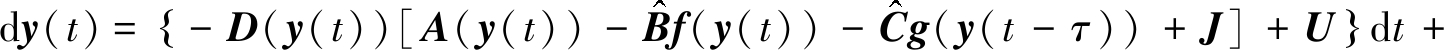H(t,y(t)-m(t)x(t),y(t-τ)-m(t-τ)x(t-τ))dW,

(2)

LV=∂V/∂t+∂V/∂x·f+(1/2)trace[σT(∂2V/∂xixj)σ],

V/∂x=(∂V/∂x1,∂V/∂x2,…,∂V/∂xn)．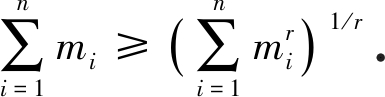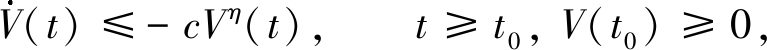V1-η(t)≤V1-η(t0)-c(1-η)(t-t0), t0tt1,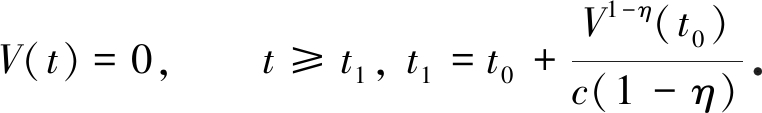## 2 控制器设计与同步分析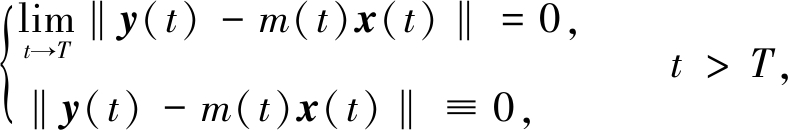(3)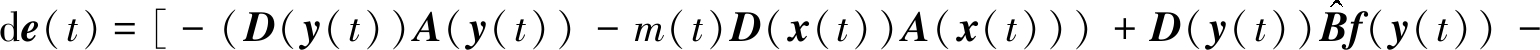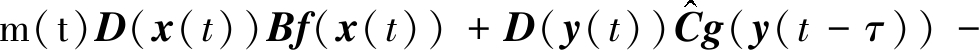m(t)D(x(t))Cg(x(t-τ))-[D(y(t))-m(t)D(x(t))]J-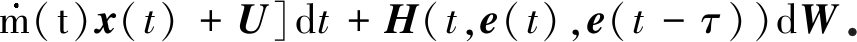(4)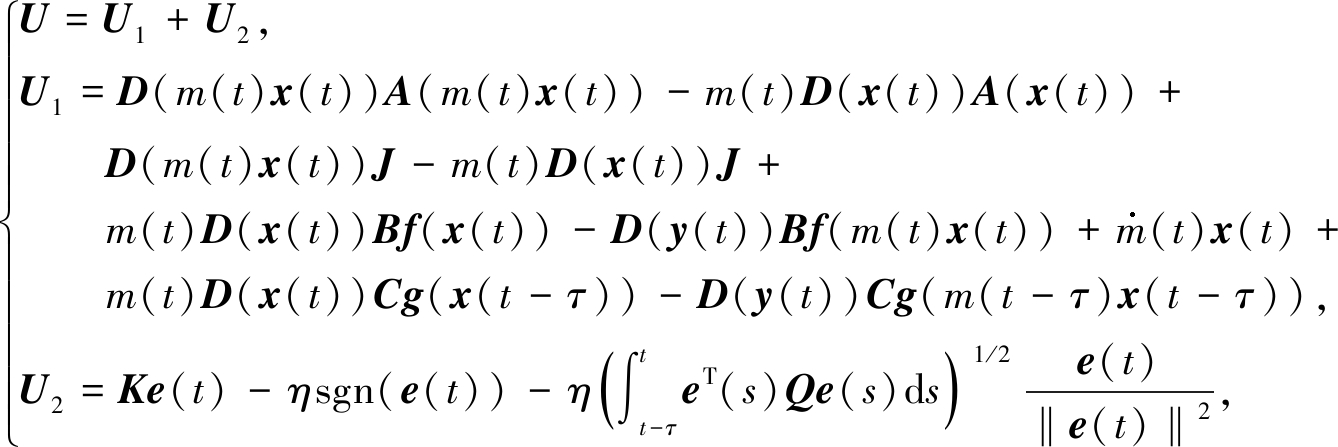(5)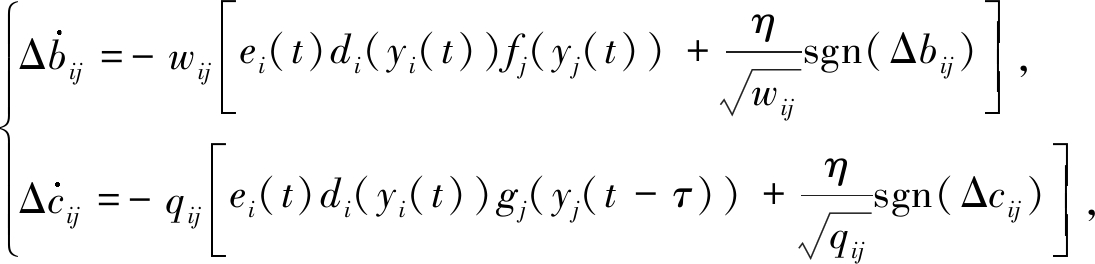(6)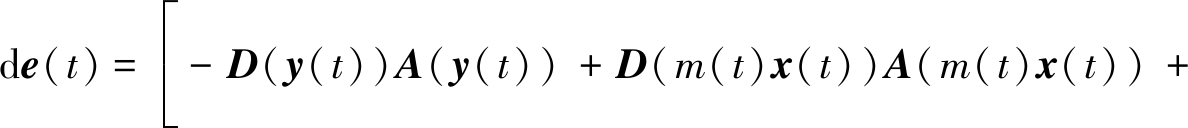D(y(t))Bf(e(t))+D(y(t))Cg(e(t-τ))+D(y(t))ΔBf(y(t))+

D(y(t))ΔCg(y(t-τ))-D(e(t))J-Ke(t)-ηsgn(e(t))-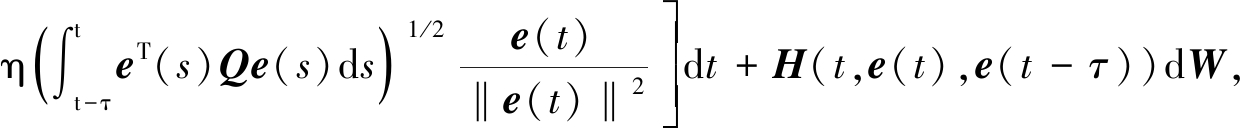(7)

f(e(t))=f(y(t))-f(m(t)x(t)),

g(e(t-τ))=g(y(t-τ))-g(m(t-τ)y(t-τ)),

D(e(t))=D(y(t))-D(m(t)x(t))．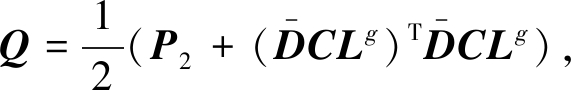(8)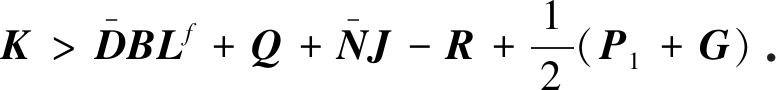(9)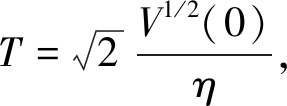(10)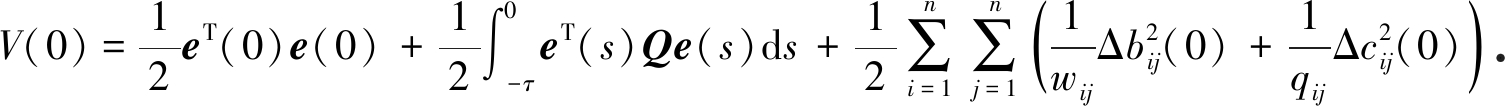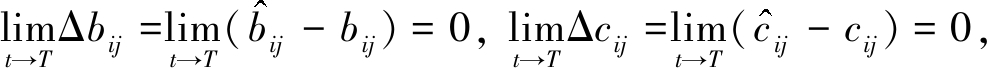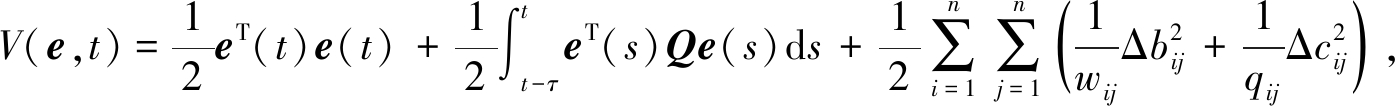(11)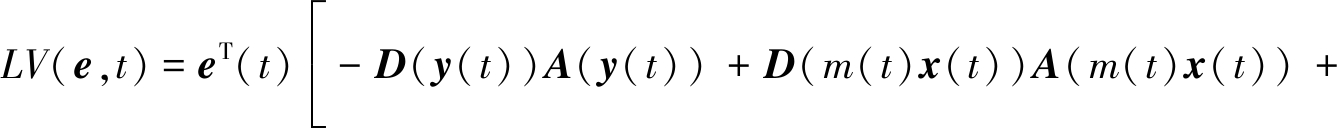D(y(t))Bf(e(t))+D(y(t))Cg(e(t-τ))-

(D(y(t))-D(m(t)x(t)))J-Ke(t)-ηsgn(e(t))-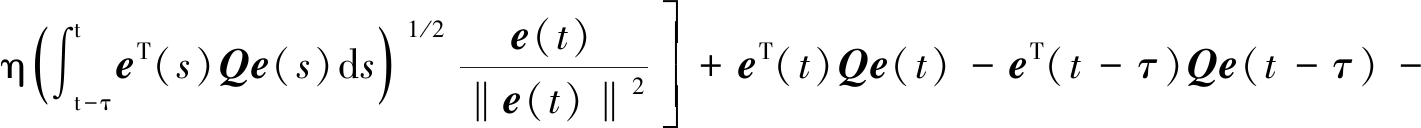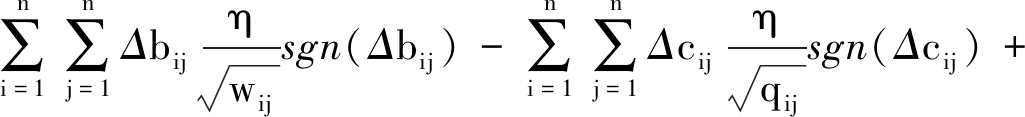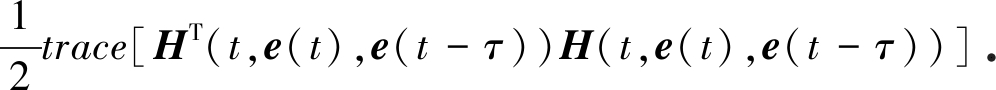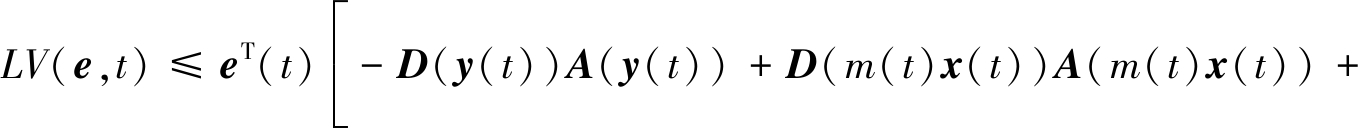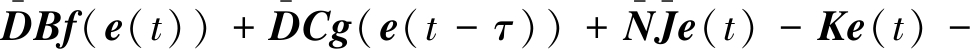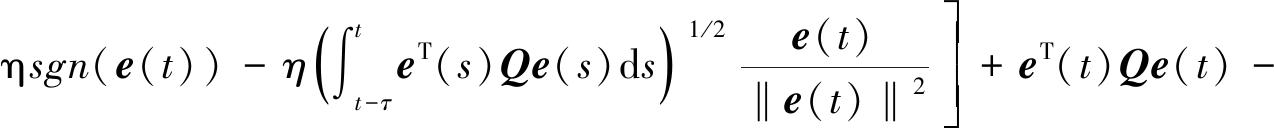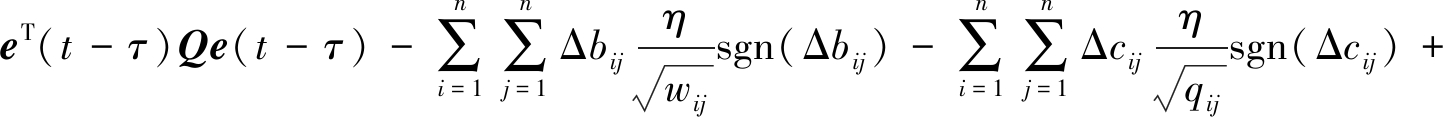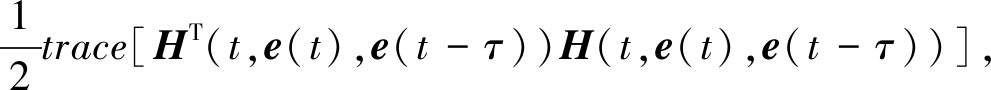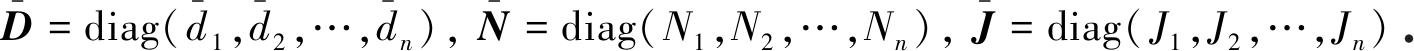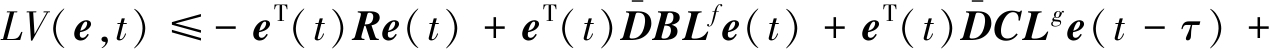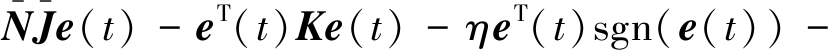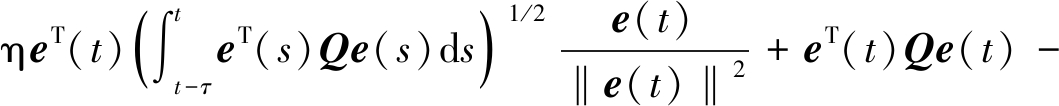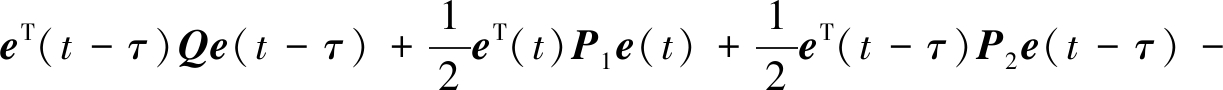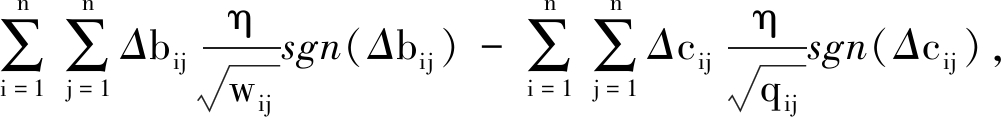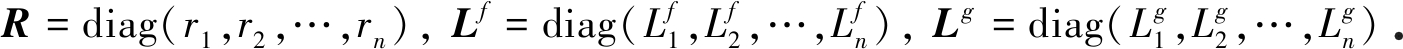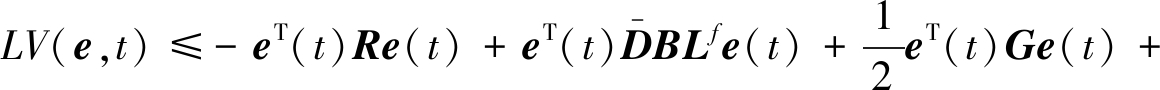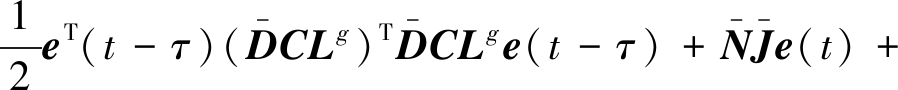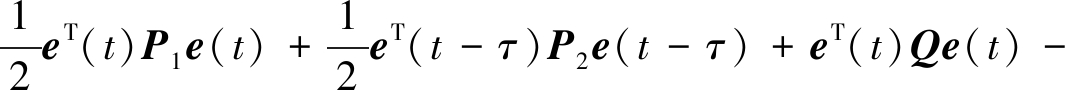eT(t-τ)Qe(t-τ)-eT(t)Ke(t)-ηeT(t)sgn(e(t))-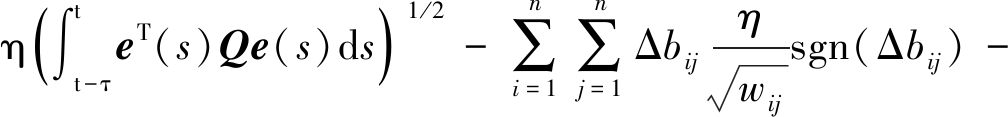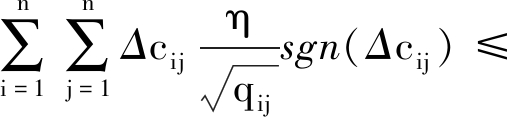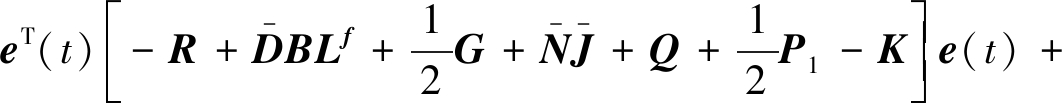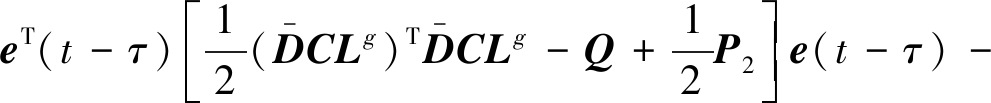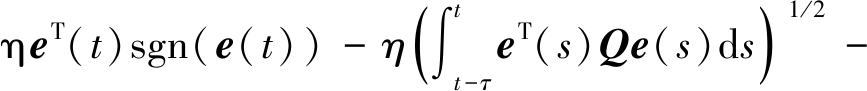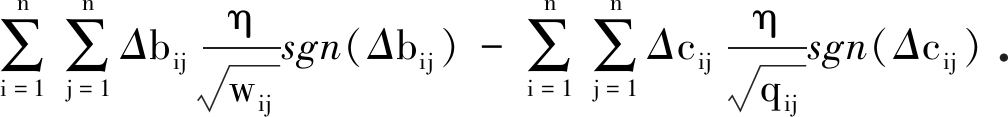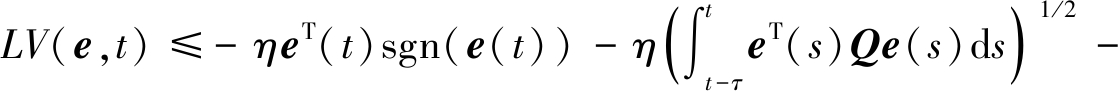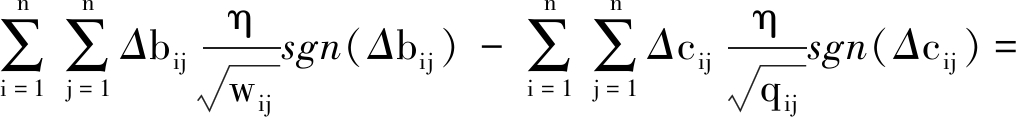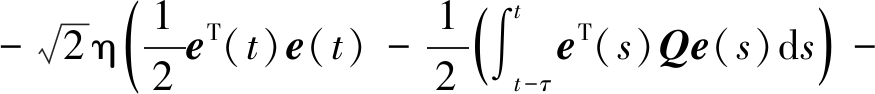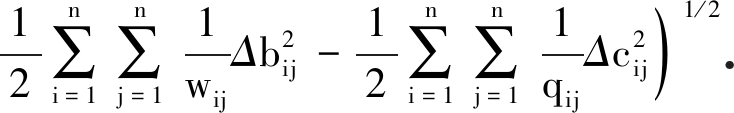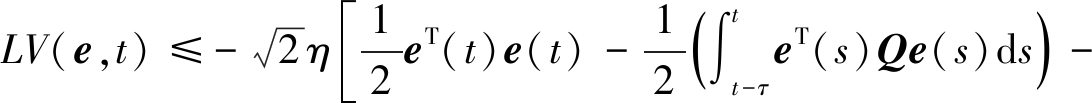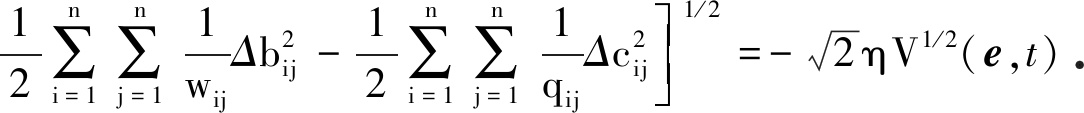dx(t)=(-A(x(t))+Bf(x(t))+Cg(x(t-τ))-J)dt

(12)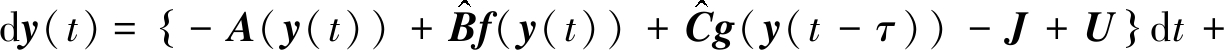H(t,y(t)-m(t)x(t),y(t-τ)-m(t-τ)x(t-τ))dW

(13)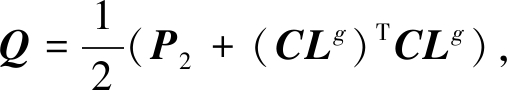(14)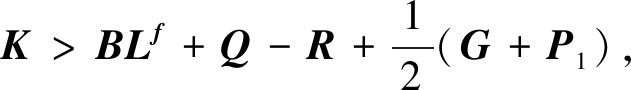(15)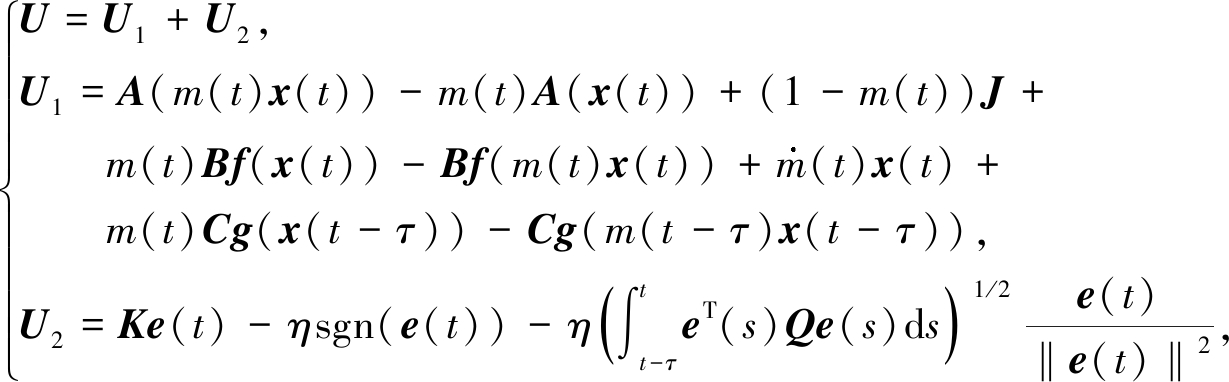(16)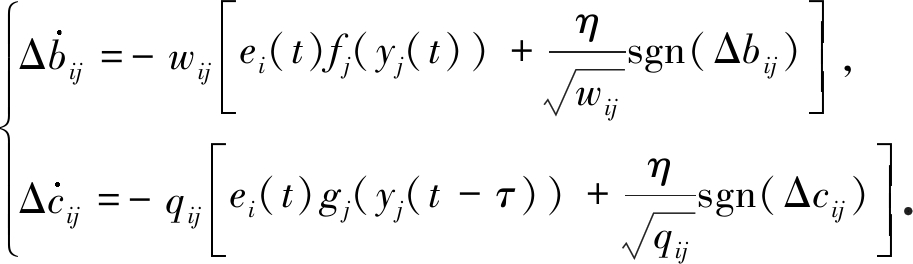(17)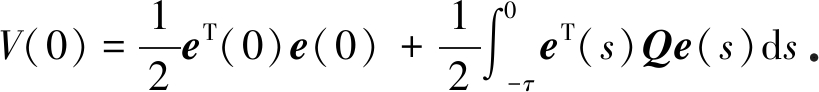## 3 数 值 仿 真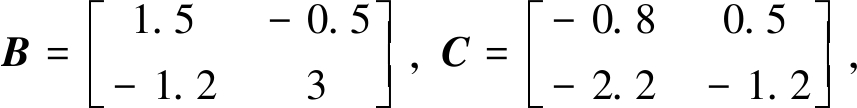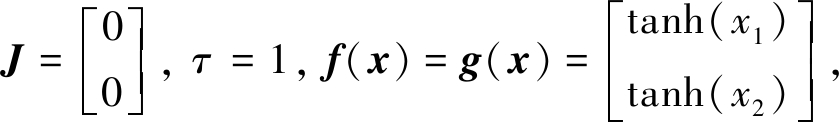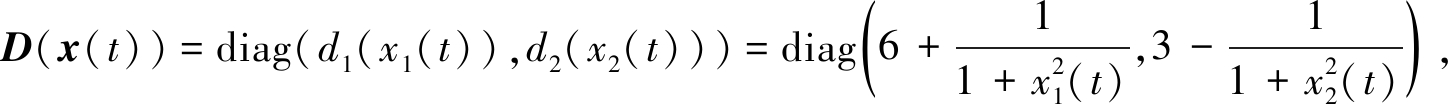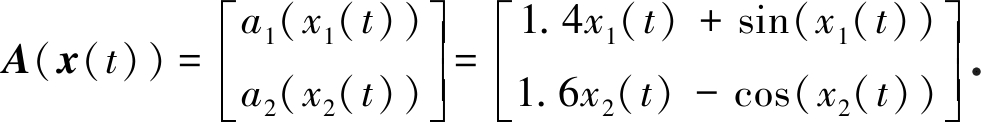H(t,e(t),e(t-τ))=h0diag(e1(t)-e1(t-τ),e2(t)-e2(t-τ))．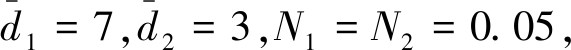所有假设均成立．根据定理1，选择η=0.1,k1=k2=20．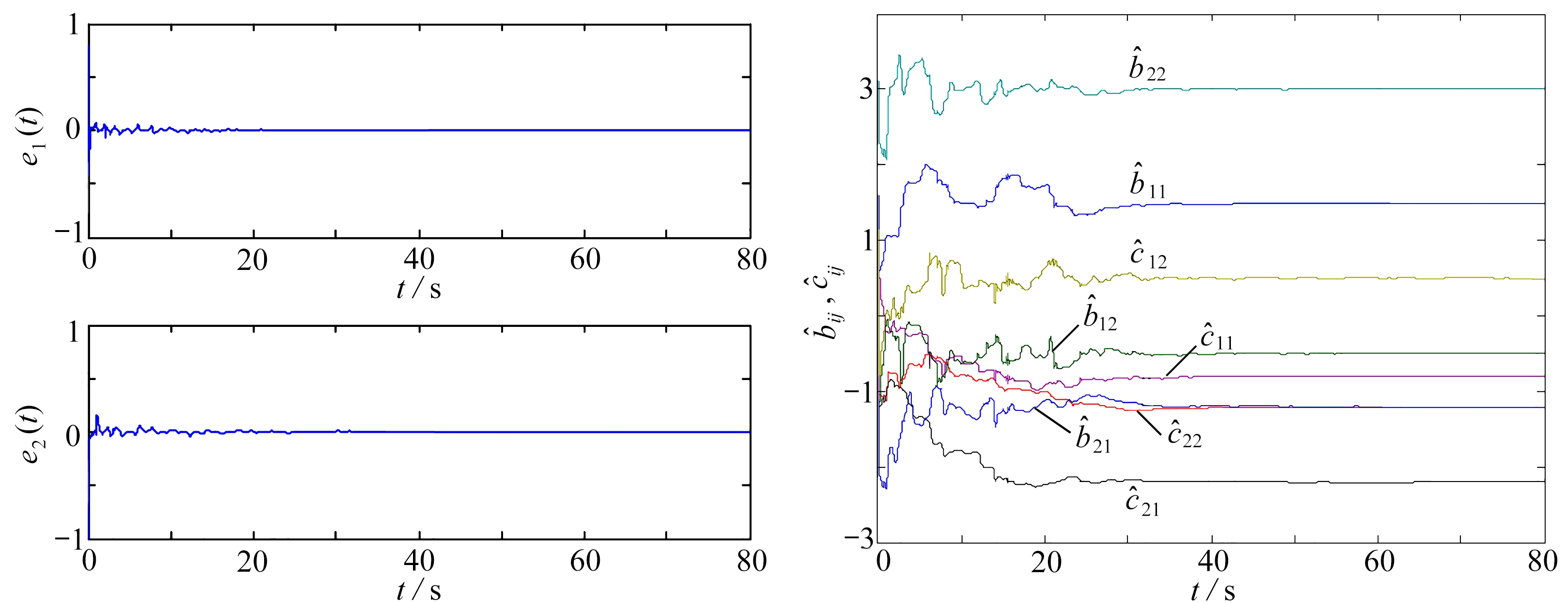Fig. 1 Time evolutions of synchronization errors Fig. 2 Dynamical behaviors of unknown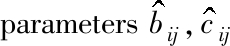## 4 保密通信应用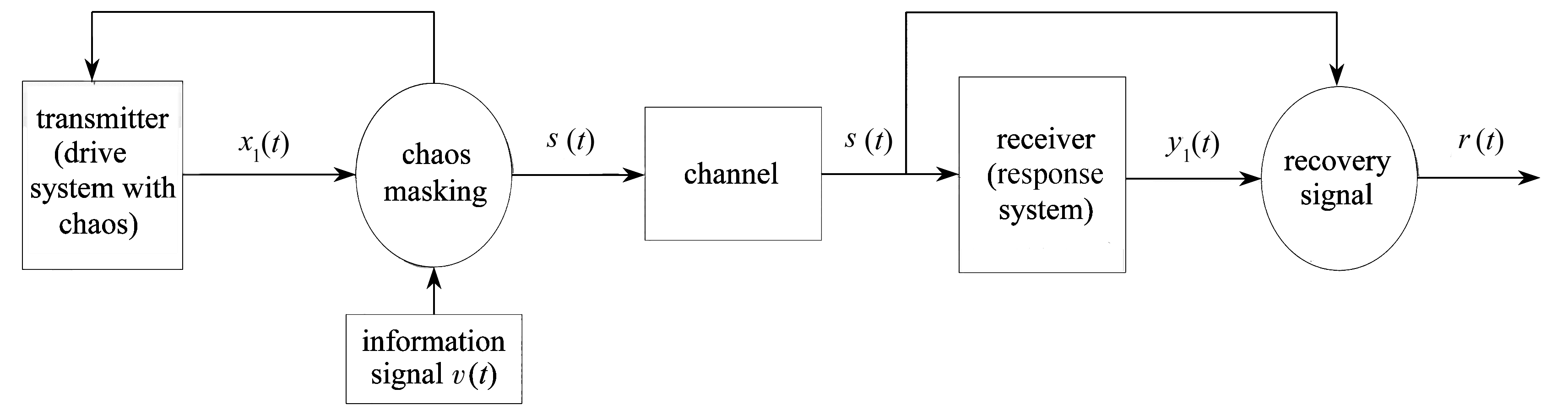Fig. 3 The secure communication system based on the finite-time function projective synchronization

dx(t)=-D(x(t))[A(x(t))-Bf(x(t))-Cg(x(t-τ))+V(t)]dt,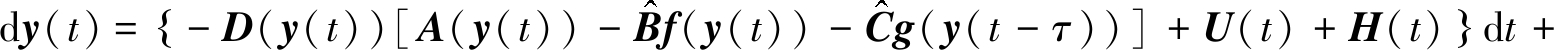H(t,e(t),e(t-τ))dW

H(t)=(-y1(t)/m(t)+s(t),-y2(t)/m(t)+x2(t))T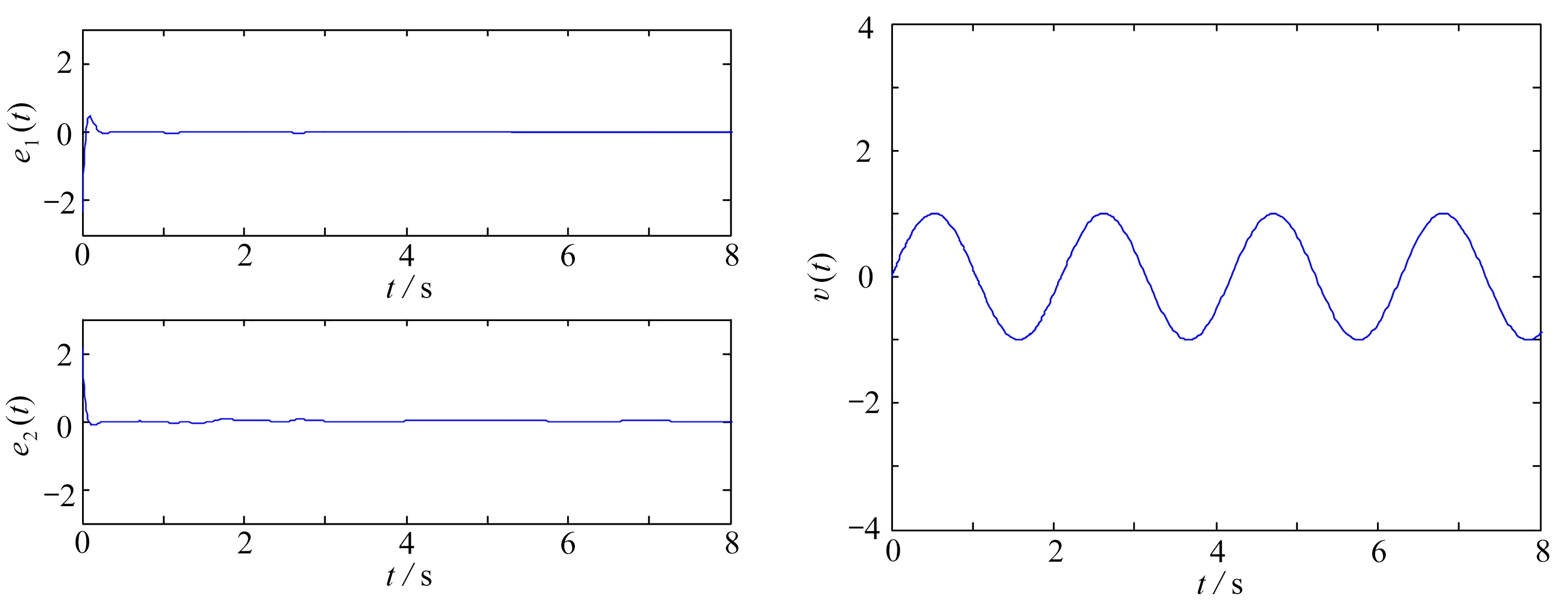(a) 同步误差 (b) 有效信号
(a) Synchronization errors (b) The information signal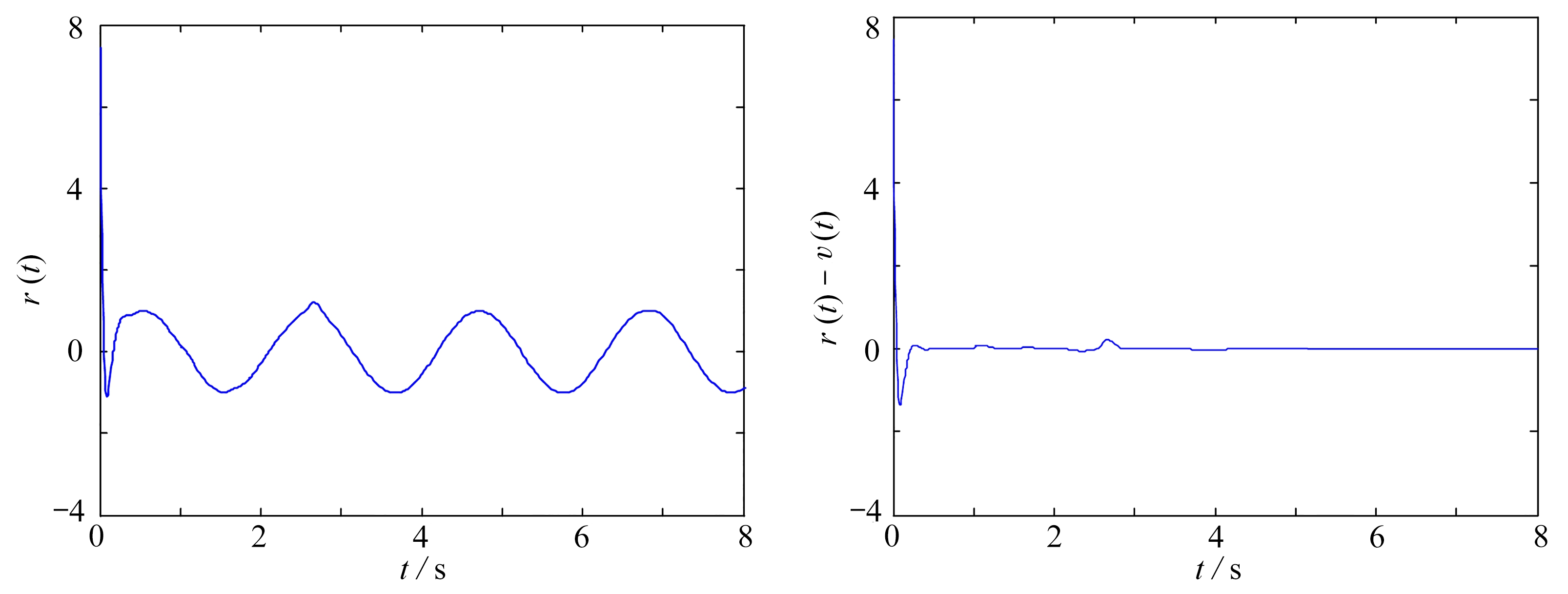(c) 被恢复的信号 (d) 有效信号与被恢复信号间的误差
(c) The recovered signal (d) The error between the information signal and the recovered signal

Fig. 4 Simulation results of recovering the information signal

## 5 结 论

 ZHANG H, WANG Z, LIU D. A comprehensive review of stability analysis of continuous-time recurrent neural networks[J]. IEEE Transactions on Neural Networks and Learning Systems, 2014, 25: 1229-1262.

 COHEN M, GROSSBERG S. Absolute stability of global pattern formation and parallel memory storage by competitive neural networks[J]. IEEE Transactions on Systems, Man and Cybernetics, 1987, 42: 288-308.

 WU Z G, SHI P, SU H Y, et al. Stochastic synchronization of Markovian jump neural networks with time-varying delay using sampled data[J]. IEEE Transactions on Cybernetics, 2013, 43: 1796-1806.

 WANG Y W, WANG H O, XIAO J W, et al. Synchronization of complex dynamical networks under recoverable attacks[J]. Automatica, 2010, 46(1): 197-203.

 WANG Z, ZHANG H. Synchronization stability in complex interconnected neural networks with nonsymmetric coupling[J]. Neurocomputing, 2013, 108: 84-92.

 韩敏, 张雅美, 张檬. 具有双重时滞的时变耦合复杂网络的牵制外同步研究[J]. 物理学报, 2015, 64(7): 070506.(HAN Min, ZHANG Yamei, ZHANG Meng. Outer synchronization analysis of two time-varying networks with double delays based on pinning control[J]. Acta Physica Sinica, 2015, 64(7): 070506.(in Chinese))

 艾合麦提·麦麦提阿吉, 李洪利. 含分布时滞递归神经网络的一般衰减同步[J]. 应用数学和力学, 2019, 40(11): 1204-1213.(MUHAMMADHAJI Ahmadjan, LI Hongli. General decay synchronization for recurrent neural networks with distributed time delays[J]. Applied Mathematics and Mechanics, 2019, 40(11): 1204-1213.(in Chinese))

 张玮玮, 陈定元, 吴然超, 等. 一类基于忆阻器分数阶时滞神经网络的修正投影同步[J]. 应用数学和力学, 2018, 39(2): 239-248.(ZHANG Weiwei, CHEN Dingyuan, WU Ranchao, et al. Modified projective synchronization of memristor-based fractional-order delayed neural networks[J]. Applied Mathematics and Mechanics, 2018, 39(2): 239-248.(in Chinese))

 JING T Y, ZHANG D Y, MEI J, et al. Finite-time synchronization of delayed complex dynamic networks via aperiodically intermittent control[J]. Journal of the Franklin Institute, 2019, 356: 5464-5484.

 LU J Y, GUO Y P, JI Y D, et al. Finite-time synchronization for different dimensional fractional-order complex dynamical networks[J]. Chaos, Solitons & Fractals, 2020, 130: 109433.

 ZHU Q X, CAO J D. Adaptive synchronization of chaotic Cohen-Crossberg neural networks with mixed time delays[J]. Nonlinear Dynamics, 2010, 61: 517-534.

 GAN Q T. Adaptive synchronization of Cohen-Grossberg neural networks with unknown parameters and mixed time-varying delays[J]. Communications in Nonlinear Science and Numerical Simulation, 2012, 17(7): 3040-3049.

 HU C, YU J, JIANG H J. Finite-time synchronization of delayed neural networks with Cohen-Grossberg type based on delayed feedback control[J]. Neurocomputing, 2014, 143: 90-96.

 LI D, CAO J D. Finite-time synchronization of coupled networks with one single time-varying delay coupling[J]. Neurocomputing, 2015, 166: 265-270.

 SHI Y C, CAO J D. Finite-time synchronization of memristive Cohen-Grossberg neural networks with time delays[J]. Neurocomputing, 2020, 377: 159-167.

 ZHANG R, YANG Y Q, XU Z Y, et al. Function projective synchronization in drive-response dynamical network[J]. Physics Letters A, 2010, 374(30): 3025-3028.

 HAN M, ZHANG Y M. Complex function projective synchronization in drive-response complex-variable dynamical networks with coupling time delays[J]. Journal of the Franklin Institute, 2016, 353(8): 1742-1758.

 SUN Y Z, ZHAO D H. Effects of noise on the outer synchronization of two unidirectionally coupled complex dynamical networks[J]. Chaos: an Interdisciplinary Journal of Nonlinear Science, 2012, 22: 023131.

 LAI Y M, PORTER M A. Noise-induced synchronization, desynchronization, and clustering in globally coupled nonidentical oscillators[J]. Physical Review E, 2013, 88(1): 012905.

 SHI Y C, ZHU P Y. Adaptive synchronization of different Cohen-Grossberg chaotic neural networks with unknown parameters and time-varying delays[J]. Nonlinear Dynamics, 2013, 73(3): 1721-1728.

 ZHOU L L, WANG C H, HE H Z, et al. Time-controllable combinatorial inner synchronization and outer synchronization of anti-star networks and its application in secure communication[J]. Communications in Nonlinear Science and Numerical Simulation, 2015, 22(1/3): 623-640.

# Finite-Time Function Projective Synchronization of Unknown Cohen-Grossberg Neural Networks With Time Delays and Stochastic Disturbances and Its Application in Secure Communication

ZHANG Yamei1, HAO Tao1, YIN Sibei1, ZHANG Meng2

(1. Department of Electrical and Automation, Shandong Labor Vocational and Technical College, Jinan 250000, P.R.China;2. School of Automation, Shenyang Aerospace University, Shenyang 110000, P.R.China)

Abstract: The finite-time function projective synchronization of unknown Cohen-Grossberg neural networks with time delays and stochastic disturbances was investigated. A hybrid control scheme combining open-loop control and feedback control was designed to guarantee that the drive and response networks can be synchronized up to a scaling function in a finite time with parameter identification by means of the finite-time stability theory. Besides, the upper bounds of the settling time of synchronization were estimated. Finally, the corresponding numerical simulation and its application in secure communication were provided to demonstrate the validity of the presented synchronization method.

Key words: synchronization; Cohen-Grossberg neural network; finite time; secure communication

DOI: 10.21656/1000-0887.410025

* 收稿日期： 2020-01-13； 修订日期：2020-11-04